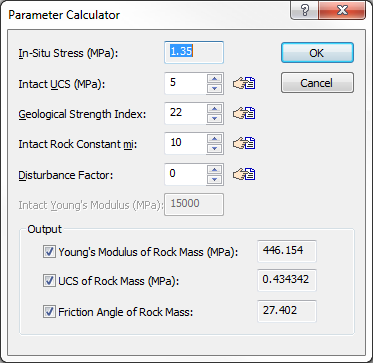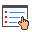Expert Modelling: Hands-on help from Rocscience Engineers. Register Here

# Parameter Calculator

The Parameter Calculator dialog allows you to calculate the following rock mass parameters:

• Young's Modulus
• Compressive Strength
• Friction Angle

from the Generalized Hoek-Brown parameters GSI, mi, D and Intact UCS. The Parameter Calculator is available for all Solution Methods, except the Carranza-Torres method, as defined in Project Settings.

This is a very useful feature of RocSupport, since the rock mass Young's Modulus, Compressive Strength, and Friction Angle are often not well known quantities, whereas the parameters GSI, mi, D and Intact UCS are more easily obtainable. For details about the calculations involved, see Hoek et. al. (2002) and Hoek and Diederichs (2005).

To use the Parameter Calculator dialog:

1. In the Tunnel and Rock Parameters dialog, select the Calculate from GSI button. This will display the Parameter Calculator dialog.1. Enter values of Intact UCS, GSI, mi and D (and Intact Modulus, if applicable, see below). The values of rock mass Young's Modulus, Compressive Strength, and Friction Angle will be automatically calculated and displayed in the dialog. NOTE:
• Whenever you see abutton beside an input edit box, if you select this button you will see another dialog, in the form of a chart or table, which allows you to estimate a suitable value for the parameter. These "assist" or "pick" dialogs are available for Intact UCS, GSI, mi and D.
• If you do not wish to have all 3 parameters calculated, you can use the check boxes to select which parameters will be calculated. This is useful if you already have known values of some parameters, which you do not wish to overwrite in the Tunnel and Rock Parameters dialog.
• Young's Modulus is estimated according to the Modulus Estimation Method selected in Project Settings. If the Modulus Estimation Method is Hoek-Diederichs (2005), then you must enter the Intact Rock Modulus in order to estimate the Rock Mass Modulus.
2. When the desired parameters have been calculated, select OK in the Parameter Calculator dialog, and the calculated values will be automatically loaded into the Tunnel and Rock Parameters dialog. Note: the values will be rounded to an appropriate number of decimal places. For example, Young's Modulus will be rounded to the nearest whole number.